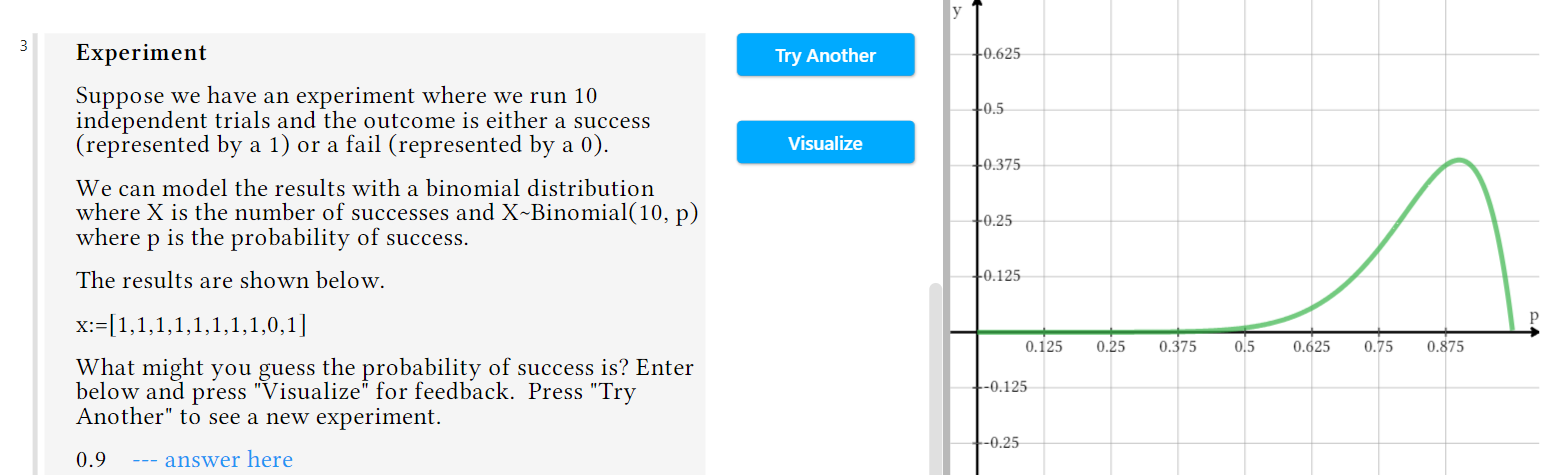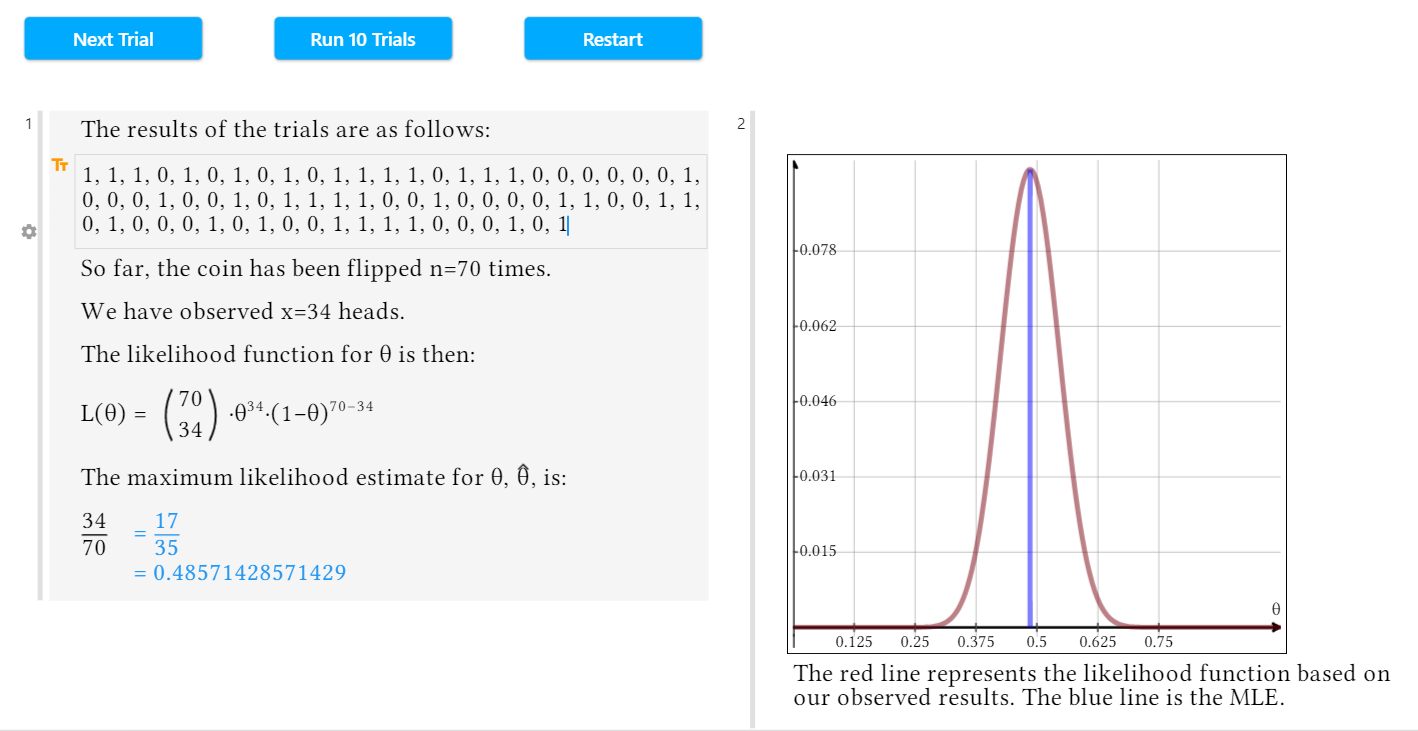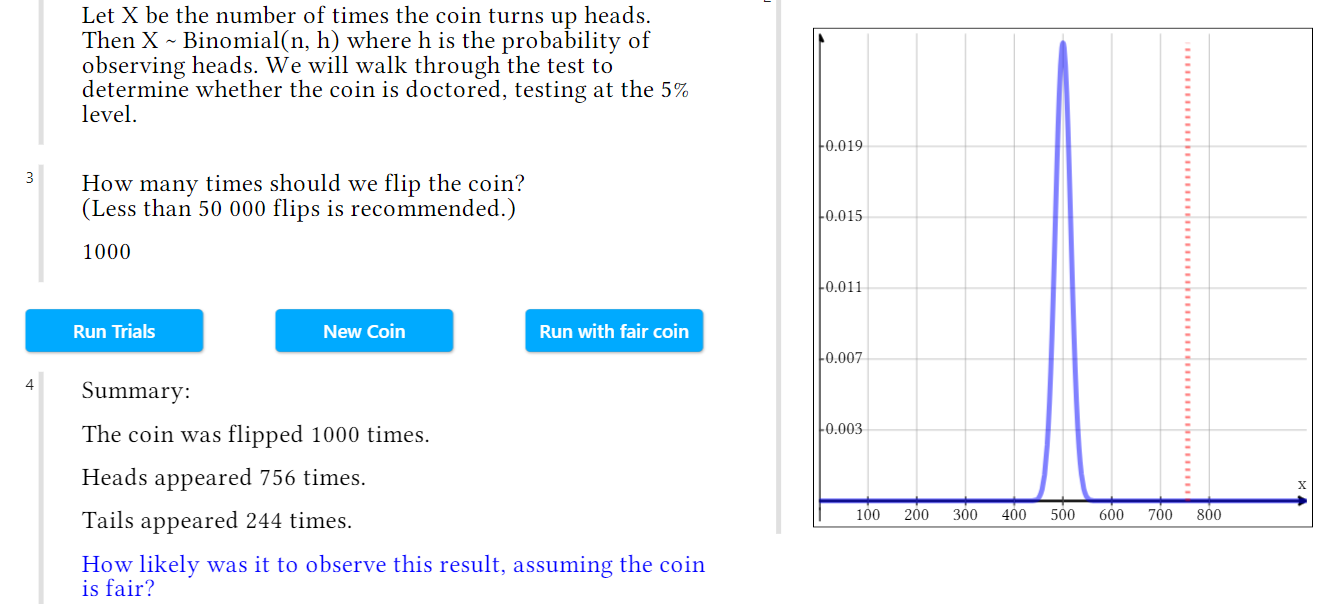## Estimation and Hypothesis Testing with Maple Learn

Maple Learn

Many everyday decisions are made using the results of coin flips and die rolls, or of similar probabilistic events. Though we would like to assume that a fair coin is being used to decide who takes the trash out or if our favorite soccer team takes possession of the ball first, it is impossible to know if the coin is weighted from a single trial.

Instead, we can perform an experiment like the one outlined in Hypothesis Testing: Doctored Coin. This is a walkthrough document for testing if a coin is fair, or if it has been doctored to favor a certain outcome.

This hypothesis testing document comes from Maple Learn’s new Estimating collection, which contains several documents, authored by Michael Barnett, that help build an understanding of how to estimate the probability of an event occurring, even when the true probability is unknown.

One of the activities in this collection is the Likelihood Functions - Experiment document, which builds an intuitive understanding of likelihood functions. This document provides sets of observed data from binomial distributions and asks that you guess the probability of success associated with the random variable, giving feedback based on your answer.Once you’ve developed an understanding of likelihood functions, the next step in determining if a coin is biased is the Maximum Likelihood Estimate Example – Coin Flip activity. In this document, you can run as many randomized trials of coin flips as you like and see how the maximum likelihood estimate, or MLE, changes, bearing in mind that if a coin is fair, the probability of either heads or tails should be 0.5.Finally, in order to determine in earnest if a coin has been doctored to favor one side over the other, a hypothesis test must be performed. This is a process in which you test any data that you have against the null hypothesis that the coin is fair and determine the p-value of your data, which will help you form your conclusion.This Hypothesis Testing: Doctored Coin document is a walkthrough of a hypothesis test for a potentially biased coin. You can run a number of trials on this coin, determine the null and alternative hypotheses of your test, and find the test statistic for your data, all using your understanding of the concepts of likelihood functions and MLEs. The document will then guide you through the process of determining your p-value and what this means for your conclusion.

So if you’re having suspicions that a coin is biased or that a die is weighted, check out Maple Learn’s Estimating collection and its activities to help with your investigation!﻿